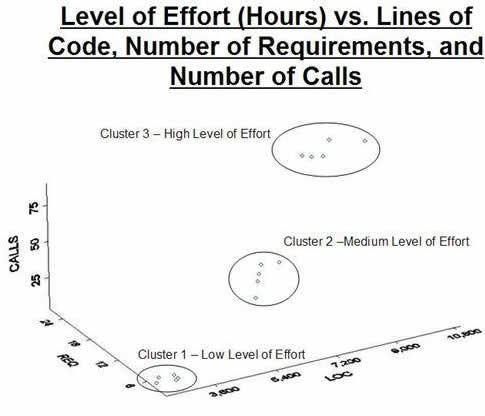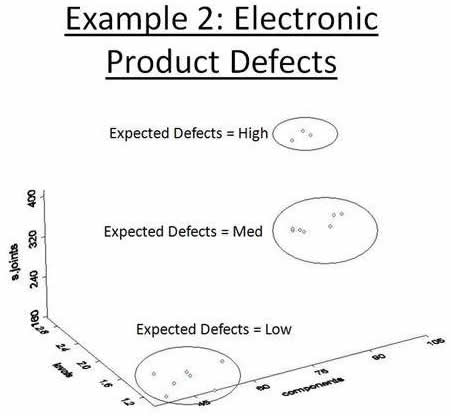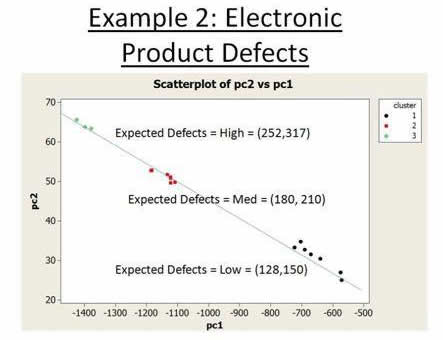Quality Digest

Featured Product
This Week in Quality Digest Live
Six Sigma Features
Cameron Shaheen
Avoid the perfect storm of motion waste, transportation waste, and time waste
David Isaacson
Best practices for conducting effective root cause analysis in manufacturing
David Cahn
Your supply chain must be agile and responsive to the changing needs of your customers
James Wells
If the solution is obvious, just do it!
Gregg Profozich
Six Sigma principles and tools
Six Sigma News
Is the future of quality management actually business management?
Too often process enhancements occur in silos where there is little positive impact on the big picture
Collect measurements, visual defect information, simple Go/No-Go situations from any online device
Good quality is adding an average of 11 percent to organizations’ revenue growth
Floor symbols and decals create a SMART floor environment, adding visual organization to any environment
A guide for practitioners and managers
Making lean Six Sigma easier and adaptable to current workplaces
Gain visibility into real-time quality data to improve manufacturing process efficiency, quality, and profits
Makes it faster and easier to find and return tools to their proper placesBio

Six Sigma

Measuring Complexity

A method for measuring the level of defects effort and development time.

Published: Tuesday, August 17, 2010 - 05:00

Complexity can be thought of as the level of difficulty in solving mathematically presented problems. Six Sigma practitioners and operations research professionals are often asked to predict the complexity of a hardware or software product by predicting (in man-hours or full-time equivalents) the expected development time, the expected number of customer-facing defects, the expected number of production defects, or the expected level of effort for a new object.

One effective approach I employ to solve this problem involves combining two statistical techniques: cluster analysis and principal component analysis. Employing cluster analysis helps to identify objects that are similar. The advantage of cluster analysis over other statistical techniques such as discriminant analysis is that the groups are determined by the cluster analysis and aren’t predetermined before the analysis. After the groups are established, employing principal component analysis, a data reduction technique, enables the practitioner to map the attributes of an object into a cluster of similar objects.

For the purposes of this discussion, the term “object” refers to hardware or a software product. Objects have properties that are called attributes, and the values we assign to an attribute represent the characteristics of the product and are referred to as “instances of the attribute.” When we have a collection of instances, we can refer to the collection of numbers as “data.”

Cluster analysis

Cluster analysis can be thought of as an aggregation of objects based on the concept of distance. A measure of distance that accounts for the similarity between objects and their relative distance is Euclidean distance. If we leverage the concept of Euclidean distance, then we can minimize the mean absolute deviation between a collection of objects from a centroid to cluster a collection of objects into groups that have common properties.

Principal component analysis

Principal component analysis is a data-reduction technique. If we describe an object based on a number of attributes (or descriptors), then we can use principal component analysis to reduce the number of attributes. Instances of the descriptors are a set of data. For example, an object that has three attributes (e.g. a person’s height, width, and girth) would have three dimensions of data. Principal component analysis gives us a way to describe that same set of characteristics using a smaller number of attributes. In the example that was just mentioned, an index value that we could call “size” might be used to characterize the height, width, and girth. Therefore, we are describing the same object in terms of one attribute rather than three.

Transformation

Mathematically, principal component analysis is a transformation. The direction of the transformation is based on the maximum variation in the data. If we have three-dimensional data then we can use principal component analysis to reduce the number of dimensions. The number of dimensions will depend on the number of directions of variation in the data, and the degree of variation in that direction.

There is one important advantage that the practitioner should be aware of when using principal component analysis: If the original data set is correlated, then the transformed data set will be uncorrelated. If the units of each attribute are different, then the transformation should be based on the correlation matrix and not the covariance matrix.

The approach

The goal is to develop a specified number of clusters in a collection of objects, understand the properties of each cluster, and then have a way of assigning a new object to one of these clusters so that we can make an educated prediction about the behavior of the new object. We can quickly make these calculations using commercial off-the-shelf software. Like any good model-building approach, we will clearly understand the question we need to answer, develop a model, and then verify and validate that the model is accurate.

The examples that follow illustrate our approach:

• Generate a predetermined number of clusters based on the similarity of the objects under consideration

• Establish an index using principal component analysis

• Establish the bounds of the index for each cluster

• Verify and validate the model

• Use the model to make predictions

Example No. 1: Estimating the time to develop a software release

For years, the software development community has tried to develop accurate models that can predict the development time of software releases. One of the many challenges is employing data that are relevant in an environment where the software development tools, process, and personnel change more rapidly than the software development time. However, if we examine smaller software releases, updates, or the production of software patches that have a relatively shorter software development time, then we can apply this technique to estimate the level of effort because the data are relevant to the problem under examination.

Software can be characterized by the number of lines of software code that can be assembled and executed, the number of calls to functions or subroutines, and the number of requirements met in a portion of code. This example in figure 1 uses fictitious data to illustrate the application of this technique to estimate the time to develop a small software release.Figure 1: Graphical representation of low, medium, and high levels of effortFigure 2: Principal component calculations

The principal component index “PC1Effort” illustrated in figure 2 is associated with the clusters in the following way:
If -3021 < PC1Effort < -2219 then the Level of Effort is associated with Cluster 1–Low Level of Effort

If -7085 < PC1Effort < -6063 then the Level of Effort is associated with Cluster 2–Medium Level of Effort

If -11155 < PC1Effort < -9940 then the Level of Effort is associated with Cluster 3–High Level of Effort

In this case, 97 percent of the cumulative value of the eigenvalues are represented by the first eigenvalue. Therefore, the three dimensions of data can be transformed into one dimension as represented in the equation above.Figure 3: View of transformed data

After the model is verified and validated against a test set of data (see figure 3), the practitioner can predict the expected level of effort in hours to develop a software release using estimates of the number of lines of code, the number of requirements, and the expected number of calls. If a calculation of PC1 was -7000, then we would estimate the level of effort to be between 160 and 190 hours of work.

Example No. 2: Estimating the number of defects from an electronic device

Electronic devices can be characterized based on the number of components, the number of electronic board levels, and the number of solder joints. In this example we will apply this technique to estimating the number of defects that occur during the production of a new electronic device. In this example, a fictitious data set represents the number of defects in the production of 100 units of various electronic products (see figure 4).Figure 4: Graphical view of electronic product defectsFigure 5: Principal component analysis

In this example, 97 percent of the cumulative value of the eigenvalues are represented in the first two eigenvalues. In figure 5, we transform the data from three dimensions into two dimensions and make estimates of the expected level of defects using the first two principal components. If PC1 was -1150 and PC2 was 50, then we would expect 180 to 210 defects in 100 production units. Figure 6 is a graphical representation of the product defects.Figure 6: View of transformed data

Conclusion

The examples above illustrate that complex relationships can be reduced to simple predictive models that give accurate estimates of product or software complexity. Although these examples are intended to demonstrate basic principles, the approach can be used on more complex data sets having a higher level of dimension. But as these simple examples illustrate, the quality practitioner who employs a combination of cluster analysis and principal component analysis has a powerful approach to develop predictive models that answer some common questions in our field.

For more on this subject, consult Finding Groups in Data by Leonard Kaufman and Peter Rousseeuw (John Wiley & Sons, 1990), chapters 1–5; and Applied Multivariate Statistical Analysis, by Richard Johnson and Dean Wichern (Prentice Hall, 1988) Chapter 8: Principle Components.

Discuss